Creating the Object

As mentioned in the other vignettes, the openEBGM package is capable of calculating $$EBGM$$ and quantile scores from the posterior distribution. openEBGM makes it easy to calculate such quantities using a class and object system. While creation of objects of class openEBGM is not necessary (see previous vignette), it provides access to methods for some common generic functions and reduces the number of function calls needed.

To create the object, we first need to calculate the hyperparameter estimates.

library(openEBGM)
data(caers)
proc <- processRaw(caers, stratify = FALSE, zeroes = FALSE)
squashed <- squashData(proc)
theta_init <- data.frame(alpha1 = c(0.2, 0.1, 0.3, 0.5, 0.2),
beta1  = c(0.1, 0.1, 0.5, 0.3, 0.2),
alpha2 = c(2,   10,  6,   12,  5),
beta2  = c(4,   10,  6,   12,  5),
p      = c(1/3, 0.2, 0.5, 0.8, 0.4))
hyper_estimate <- autoHyper(squashed, theta_init = theta_init,
zeroes = FALSE, squashed = TRUE, N_star = 1)

Once we have the hyperparameter estimates and the processed data, we can calculate the $$EBGM$$ scores and any desired quantile(s) from the posterior distribution.

ebout <- ebScores(proc, hyper_estimate = hyper_estimate,
quantiles = c(5, 95)) #For the 5th and 95th percentiles
ebout_noquant <- ebScores(proc, hyper_estimate = hyper_estimate,
quantiles = NULL) #For no quantiles

As seen above, we can calculate the $$EBGM$$ scores with or without adding quantiles. If using quantiles, we can specifying any number of quantiles.

Using the Generic Functions

Once the object has been created, we can use class-specific methods for some of R’s generic functions (namely, print(), summary(), and plot()).

#We can print an openEBGM object to get a quick look at the contents
print(ebout)
#>
#> There were 131 var1-var2 pairs with a QUANT_05 greater than 2
#>
#> Top 5 Highest QUANT_05 Scores
#>                                           var1                        var2
#> 13924                            REUMOFAN PLUS            WEIGHT INCREASED
#> 8187  HYDROXYCUT REGULAR RAPID RELEASE CAPLETS          EMOTIONAL DISTRESS
#> 13886                            REUMOFAN PLUS                    IMMOBILE
#> 7793              HYDROXYCUT HARDCORE CAPSULES CARDIO-RESPIRATORY DISTRESS
#> 8220  HYDROXYCUT REGULAR RAPID RELEASE CAPLETS                      INJURY
#>        N          E QUANT_05
#> 13924 16 0.40643623    15.68
#> 8187  19 0.89690107    11.65
#> 13886  6 0.07866508    10.16
#> 7793   8 0.30482718     8.99
#> 8220  11 0.56317044     8.98
print(ebout_noquant, threshold = 3)
#>
#> There were 556 var1-var2 pairs with an EBGM score greater than 3
#>
#> Top 5 Highest EBGM Scores
#>                                                     var1
#> 13924                                      REUMOFAN PLUS
#> 13886                                      REUMOFAN PLUS
#> 8187            HYDROXYCUT REGULAR RAPID RELEASE CAPLETS
#> 4093  EMERGEN-C (ASCORBIC ACID, B-COMPLEX, ELECTROLYTE,
#> 7832                        HYDROXYCUT HARDCORE CAPSULES
#>                     var2  N          E  EBGM
#> 13924   WEIGHT INCREASED 16 0.40643623 23.26
#> 13886           IMMOBILE  6 0.07866508 18.28
#> 8187  EMOTIONAL DISTRESS 19 0.89690107 16.78
#> 4093               COUGH  6 0.14481526 16.03
#> 7832   MULTIPLE INJURIES  5 0.09237187 15.63

When quantiles are present, simply printing the object shows, by default, how many var1-var2 pairs exist that have QUANT$$>x$$, where $$x$$ is the minimum quantile threshold used for the data (default 2). In the absence of quantiles, it simply outputs the number of var1-var2 pairs that have an $$EBGM$$ score greater than the specified threshold. In both cases, it also shows a quick look at the var1-var2 pairs with the highest $$x$$ or $$EBGM$$, depending on whether quantiles were calculated or not.

One can also use the summary() function on an openEBM object to get further information about the calculations.

summary(ebout)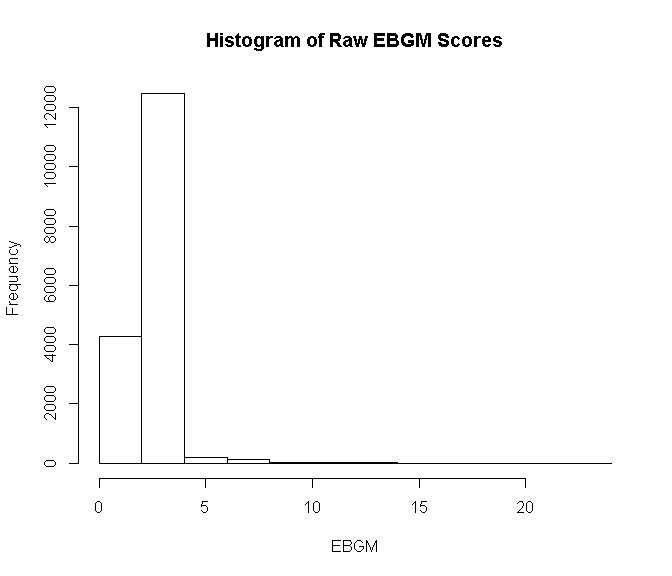#>
#> Summary of the EB-Metrics
#>       EBGM           QUANT_05          QUANT_95
#>  Min.   : 0.200   Min.   : 0.0700   Min.   : 0.51
#>  1st Qu.: 2.010   1st Qu.: 0.4800   1st Qu.:12.19
#>  Median : 2.390   Median : 0.5100   Median :14.73
#>  Mean   : 2.356   Mean   : 0.5377   Mean   :13.31
#>  3rd Qu.: 2.580   3rd Qu.: 0.5200   3rd Qu.:15.73
#>  Max.   :23.260   Max.   :15.6800   Max.   :33.48

As seen above, by default the summary() function, when called on an openEBGM object, outputs some descriptive statistics on the $$EBGM$$ and quantile scores, and a histogram of the $$EBGM$$ scores. There are options to disable plot output, or to calculate the log2 transform of the scores, which provides a Bayesian information statistic (when applied to the $$EBGM$$ score).

summary(ebout, plot.out = FALSE, log.trans = TRUE)
#>
#> Summary of the EB-Metrics
#>       EBGM           QUANT_05          QUANT_95
#>  Min.   :-2.322   Min.   :-3.8365   Min.   :-0.9714
#>  1st Qu.: 1.007   1st Qu.:-1.0589   1st Qu.: 3.6076
#>  Median : 1.257   Median :-0.9714   Median : 3.8807
#>  Mean   : 1.161   Mean   :-0.9833   Mean   : 3.6316
#>  3rd Qu.: 1.367   3rd Qu.:-0.9434   3rd Qu.: 3.9754
#>  Max.   : 4.540   Max.   : 3.9709   Max.   : 5.0652

Finally, openEBGM provides a method for the plot() function that can produce a variety of different plots. These are shown below.

plot(ebout)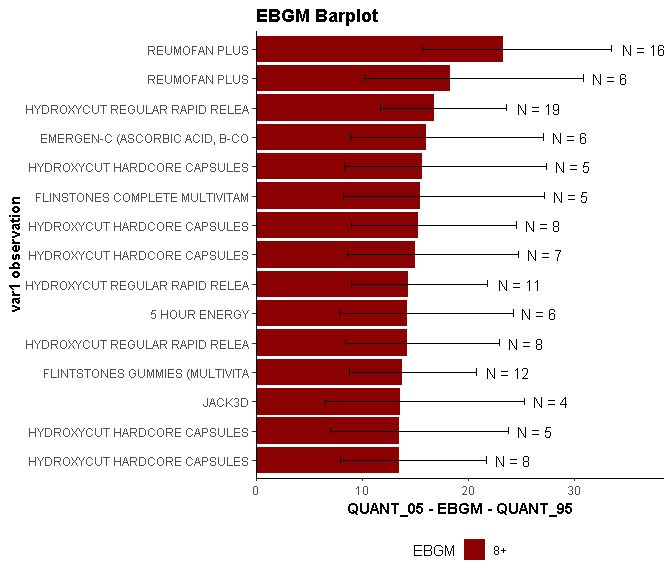As seen, by default, the plot() function shows the top $$EBGM$$ scores by var1-var2 combinations (only var1 is shown for space preservation) and “error bars” using the lowest and highest quantiles calculated. The sample size for each var1-var2 combination is also plotted.

A specific event from var2 may also be selected, and only the var1-var2 combinations that include this particular event will be shown. An example is shown below.

plot(ebout, event = "CHOKING")
#> Warning in plot.openEBGM(ebout, event = "CHOKING"): 2 or more matches found
#> for event specified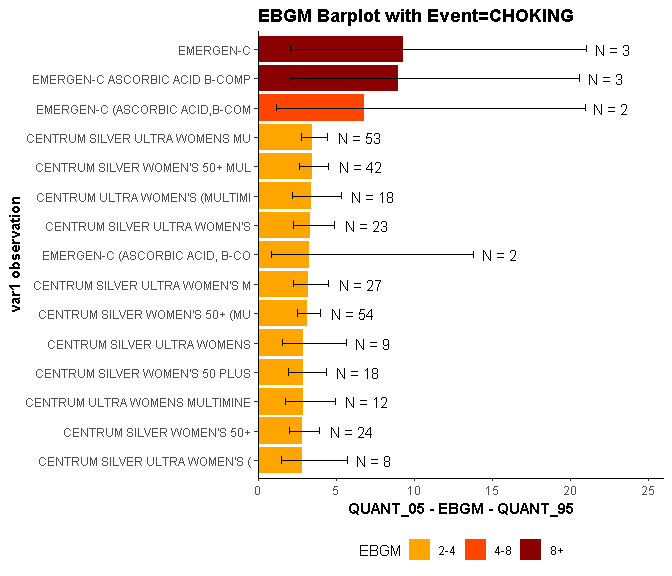In addition to the bar chart, the plot() function can also create a histogram of the $$EBGM$$ scores.

plot(ebout, plot.type = "histogram")
#> stat_bin() using bins = 30. Pick better value with binwidth.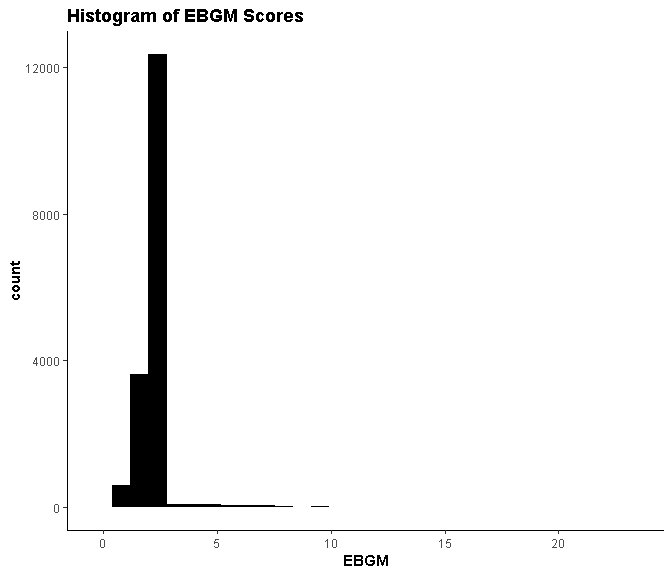Again, one may choose an event from var2 by which to subset the data when plotting.

plot(ebout, plot.type = "histogram", event = "CHOKING")
#> stat_bin() using bins = 30. Pick better value with binwidth.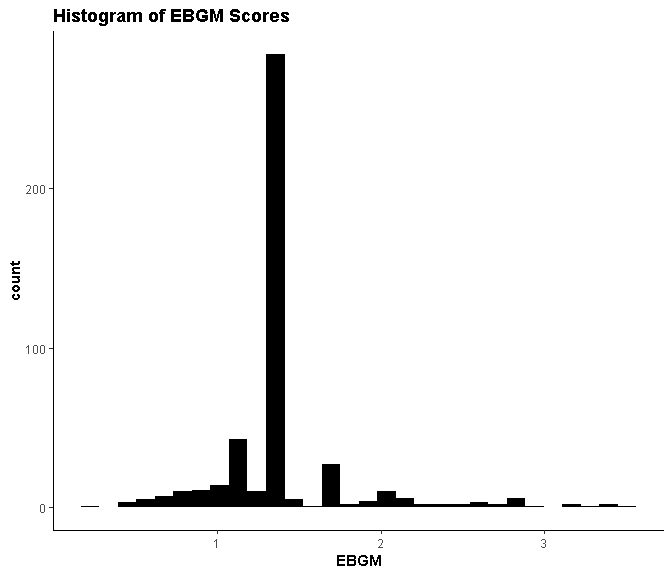Finally, the last type of plot included with the plot() function shows the shrinkage performed by the algorithm. It is called the “Chirtel Squid Plot”, titled after its creator, Stuart Chirtel.

plot(ebout, plot.type = "shrinkage")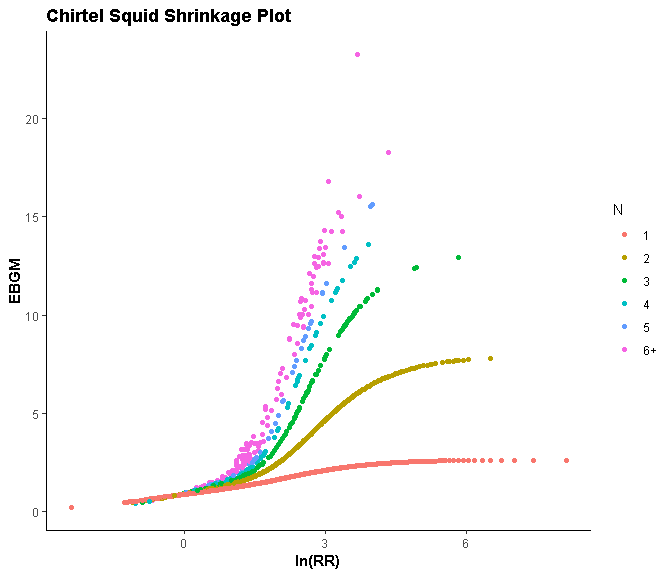While a specific event may be selected by which to subset the data, it can lead to a less informative plot due to smaller sample size.

plot(ebout, plot.type = "shrinkage", event = "CHOKING")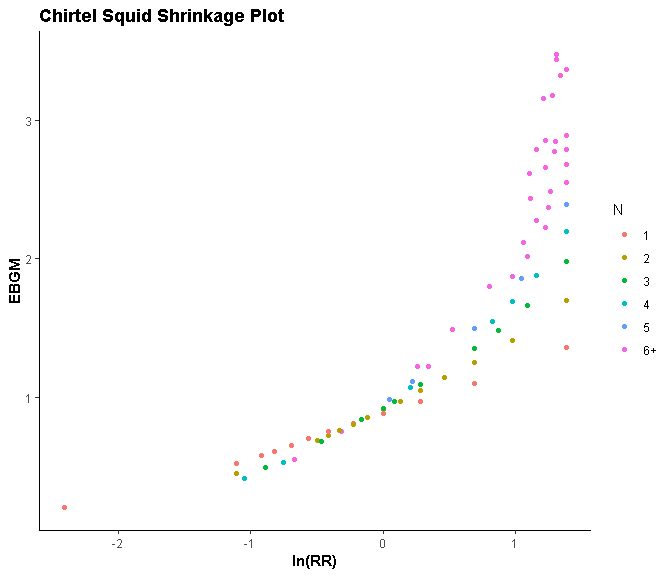Conclusion

openEBGM was designed to give the user a high level of control over data analysis choices (stratification, data squashing, etc.) using DuMouchel’s Gamma-Poisson Shrinkage (GPS) method (1999, 2001). The GPS method applies to any large contingency table, so openEBGM can be used to mine a variety of databases in which the rate of co-occurrence of two variables or items is of interest (sometimes known as the “market basket problem”). U.S. FDA products and adverse events is just one of many possible applications.